## Monday, November 26, 2018

### Principal Component Analysis: Part II (Practice)

In Part I of our series on Principal Component Analysis (PCA), we covered a theoretical overview of fundamental concepts and disucssed several inferential procedures. Here, we aim to complement our theoretical exposition with a step-by-step practical implementation using EViews. In particular, we are motivated by a desire to apply PCA to some dataset in order to identify its most important features and draw any inferential conclusions that may exist. We will proceed in the following steps:
1. Summarize and describe the dataset under consideration.
2. Extract all principal (important) directions (features).
3. Quantify how much variation (information) is explained by each principal direction.
4. Determine how much variation each variable contributes in each principal direction.
5. Reduce data dimensionality.
6. Identify which variables are correlated and which correlations are more principal.
7. Identify which observations are correlated with which variables.
The links to the workfile and program file can be found at the end.

### Principal Component Analysis of US Crime Data

We will use PCA to study US crime data. In particular, our dataset summarizes the number of arrests per 100,000 residents in each of the 50 US states in 1973. The data contains four variables, three of which pertain to arrests associated with (and naturally named) MURDER, ASSAULT, and RAPE, whereas the last, named URBANPOP, contains the percentage of the population living in urban centers.

#### Data Summary

To understand our data, we will first create a group object with the variables of interest. We can do this by selecting all four variables in the workfile by clicking on each while holding down the Ctrl button, right-clicking on any of the highlighted variables, moving the mouse pointer over Open in the context menu, and finally clicking on as Group. This will open a group object in a spreadsheet with the four variables placed in columns. The steps are reproduced in Figures 1a and 1b.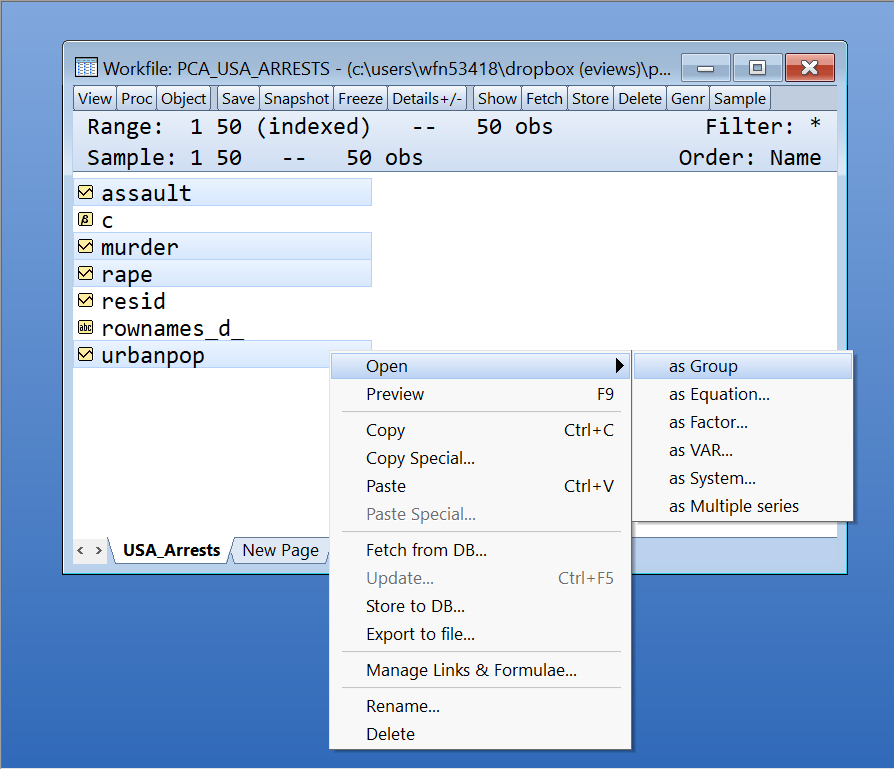Figure 1A: Open Group Figure 1B: Group Window
From here, we can derive the usual summary statistics by clicking on View in the group window, moving the mouse over Descriptive Stats and clicking on Common Sample. This produces a spreadsheet with various statistics of interest. We reproduce the steps and output in Figures 2a and 2b.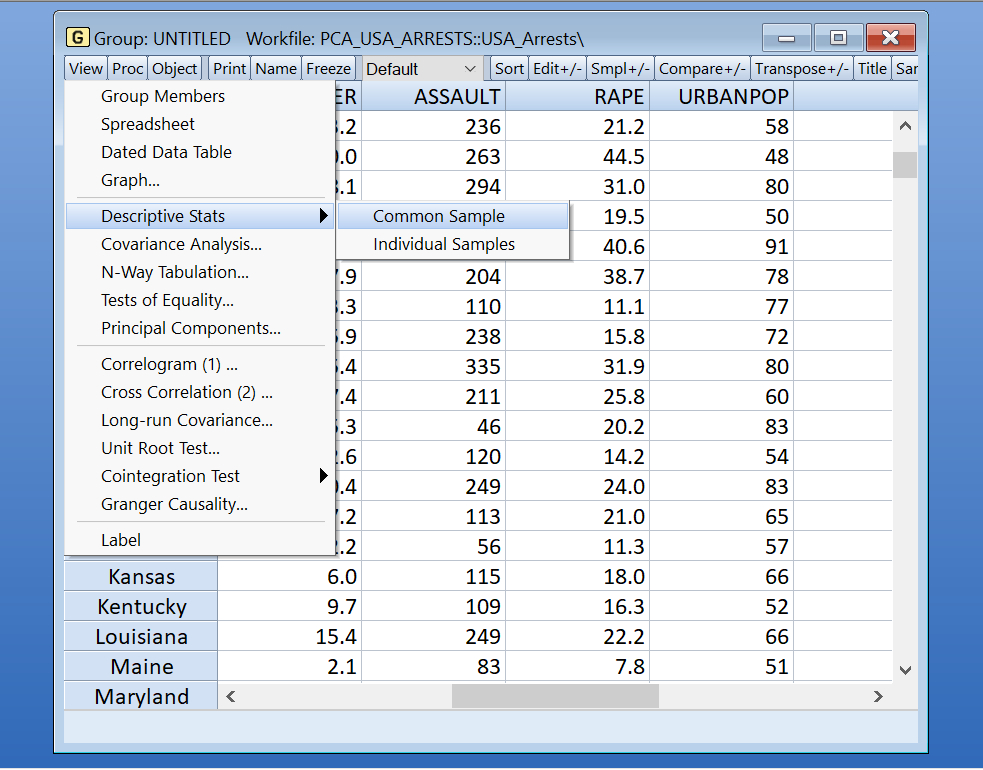Figure 2A: Descriptive Stats Menu Figure 2B: Descriptive Stats Output
We can also plot each of the series to get a better visual sense for the data. In particular, from the group window, click on View and click on Graph. This brings up the Graph Options window. Here, from the Multiple Series dropdown menu, select Multiple Graphs and click on OK. We summarize the sequence in Figures 3a and 3b.Figure 3A: Graph Options Figure 3B: Multiple Graphs
At last, we can get a sense for information redundancy (see section Variance Decomposition in Part I of this series) by studying correlation patterns. In this regard, we can produce a correlation matrix by clicking on View in the group window and clicking on Covariance Analysis.... This opens a window with further options. Here, deselect (click) the checkbox next to Covariance and select (click) the box next to Correlation. This ensures that EViews will only produce the correlation matrix without any other statistics. Furthermore, in the Layout dropbox, select Single table, and finally click on OK. Figures 4a and 4b reproduce these steps.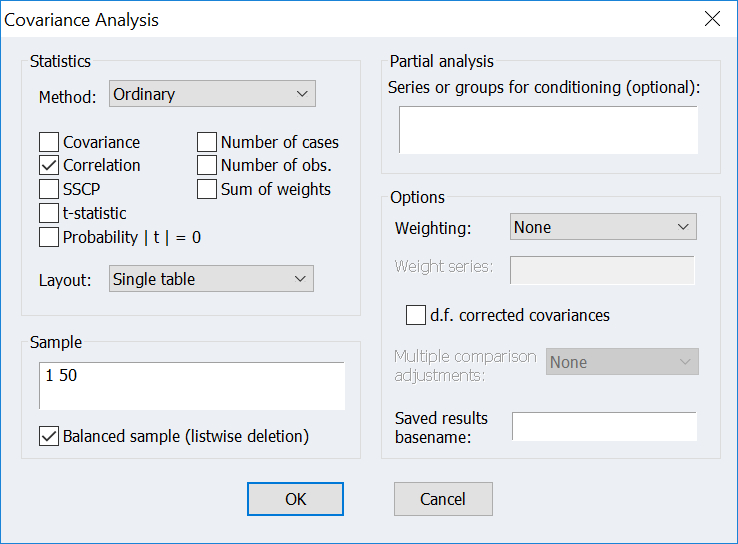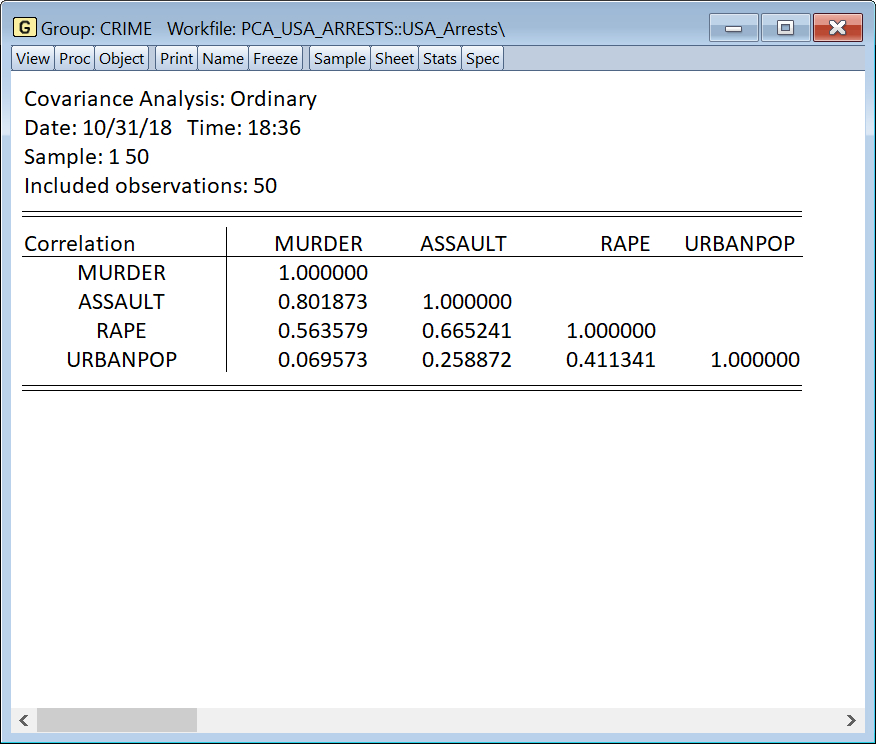Figure 4A: Covariance Analysis Figure 4B: Correlation Table
A quick interpretation of the correlation structure indicates that murder is highly correlated with assault, whereas the latter exhibits a strong positive correlation with rape. Moreover, whereas murder is nearly uncorrelated with larger urban centers, among the three causes for arrest, rape generally favours larger communities. Intuitively, this is in line with conventional wisdom. Murders are rarely observed on professional levels and typically involve assault as a precursor. Furthermore, due to higher costs of crime visibility and cleanup, murder generally does not favour larger population areas where police presence and witness visibility is generally more pronounced. On the other hand, rape favours larger urban centers due to the fact that there are simply more people and the cost to covering or denying the crime is notoriously very low. Furthermore, victims of rape in smaller communities are typically shamed into staying quiet since connection circles are naturally tighter in such surroundings.

### Principal Component Analysis of Crime Data

Doing PCA in EViews is trivial. From our group object window, click on View and click on Principal Components.... This opens the main PCA dialog. See Figure 5a and 5b below.Figure 5A: Initiating the PCA dialog Figure 5B: Main PCA Dialog
From here, EViews offers users the ability to apply several tools and protocols readily encountered in the literature on PCA.

#### Summary of Fundamentals

As a first step, we are interested in summarizing PCA fundamentals. In particular, we seek an overview of eigenvalues and eigenvectors that result from applying the principal component decomposition to the covariance or correlation matrix associated with our variables of interest. To do so, consider the Display group, and select Table. The latter produces three tables summarizing the covariance (correlation) matrix, and the associated eigenvectors and eigenvalues.

Associated to this output are several important options under the Component selection group. These include:
• Maximum number: This defaults to the theoretical maximum number of eigenvalues possible, which is the total number of variables in the group under consideration. In our case, this number is 4.
• Minimum eigenvalue: This defaults to 0. Nevertheless, selecting a positive value requests that all eigenvectors associated with eigenvalues less than this value are not displayed.
• Cumulative proportion: This defaults to 1. Choosing a value $\alpha < 1$ however, requests that only the most principal $k$ eigenvalues and eigenvectors associated with explaining $\alpha*100 \%$ of the variation are retained. Naturally, choosing $\alpha=1$ requests that all eigenvalues are displayed. See section Dimension Reduction in Part I of this series for further details.
Since we are interested in a global summary, we will leave the Component selection options at their default values.

Furthermore, consider momentarily the Calculation tab. Here, the Type dropdown offers the choice to apply the principal component decomposition either to the correlation or covariance matrix. For details, see sections Variance Decomposition and Change of Basis in Part I of this series. The choice essentially reduces to whether or not the variables under consideration exhibit similar scales. In other words, if variances of the underlying variables of interest are similar, then conducting PCA on the covariance matrix is certainly justified. Nevertheless, if the variances are widely different, then selecting the correlation matrix is more appropriate if interpretability and comparability are desired. EViews errs on the side of caution and defaults to using the correlation matrix. Since the table of summary statistics we produced in figure 3b clearly shows a lack of uniformity in standard deviations across the four variables of interest, we will stick with the default and use the correlation matrix. Hit OK.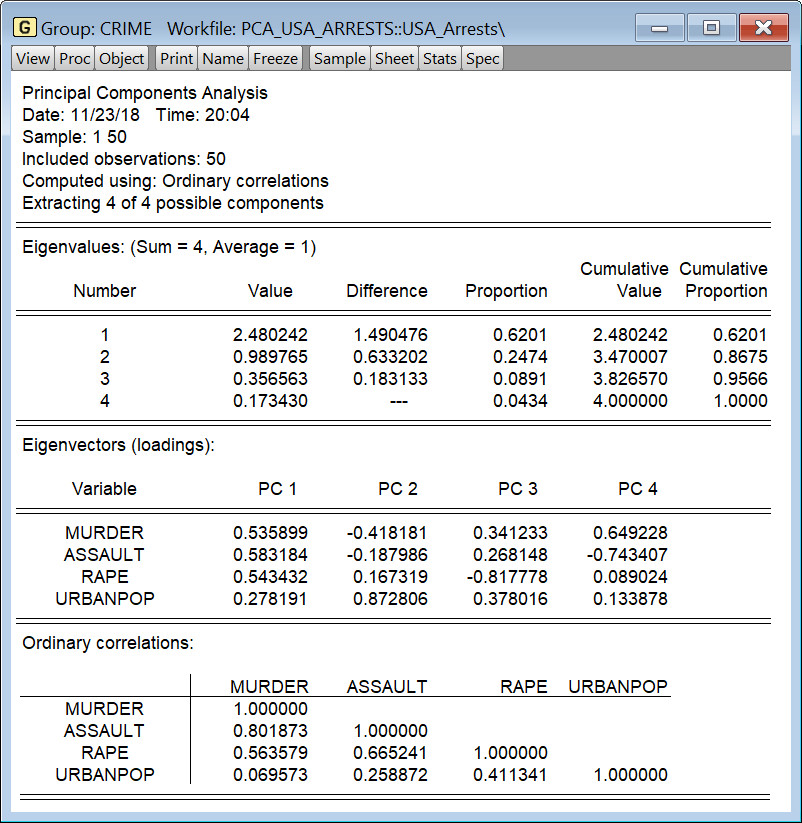Figure 6: PCA Table Output

The resulting output, which is summarized Figure 6 above, consists of three tables. The first table summarizes the information on eigenvalues. The latter are sorted in order of principality (importance), measured as the proportion of information explained by each principal direction. Refer to section Principal Directions in Part I of this series for more details. In particular, we see that the first principal direction explains roughly 62% of the information contained in the underlying correlation matrix, the second, roughly 25%, and so on. Furthermore, the cumulative proportion of information explained by the first two principal directions is roughly 87(62 + 25)%. In other words, if dimensionality reduction is desired, our analysis indicates that we can half the underlying dimensionality of the problem from 4 to 2, while retaining nearly 90% of the original information. This is evidently a profitable trade-off. For theoretical details, see section Dimension Reduction in Part I of this series. At last, observe that EViews reports that the average of the 4 eigenvalues is 1. This will in fact always be the case when extracting eigenvalues from a correlation matrix.

The second (middle) table summarizes the eigenvectors associated with each of the principal eigenvalues. Naturally, the eigenvectors are also arranged in order of principality. Furthermore, whereas the eigenvalues highlight how much of the overall information is extracted in each principal direction, the eigenvectors reveal how much weight each variable has in each direction.

Recall from Part I of this series that all eigenvectors have length unity. Accordingly, the relative importance of any variable in a given principal direction is effectively the proportion of the eigenvector length (unity) attributed to that variable. For instance, in the case of the first eigenvector, $[0.535899, 0.583184, 0.543432, 0.278191]^{\top}$, MURDER accounts for $0.535899^{2} \times 100\% = 0.287188\%$ of the overall direction length. Similarly, ASSAULT accounts for 0.340103% of the direction, and RAPE contributes 0.295318%. Evidently, the least important variable in the first principal direction is URBANPOP, which accounts for only 0.077390% of the direction length.

On the other hand, in the second principal direction, it is URBANPOP that carries most weight, contributing $0.872806 \times 100\% = 0.761790\%$ to the direction length. Accordingly, if feature extraction is the goal, it is clear (and rather obvious) that the first principal direction is roughly equally dominated by MURDER, ASSAULT, and RAPE, whereas the second principal direction is almost entirely governed by URBANPOP. For a theoretical exposition, see section Principal Components in Part I of this series.

At last, the third table is just the correlation matrix to which the eigen-decomposition is applied. The latter, while important, is provided only as a reference.

#### Eigenvalue Plots and Dimensionality

Now that we have a rough picture of PCA fundamentals associated with our dataset, it is natural to ask whether we can proceed with dimensionality reduction in a more formal manner. One such way (albeit arbitrary, but widely popular) is to look at several eigenvalue plots and visually identify how many eigenvalues to retain.

From the previous PCA output, click again on View, then Principal Components..., and select Eigenvalue Plots under the Display group. This is summarized in Figure 7 below.Figure 7: PCA Dialog: Eigenvalue Plots

Here, EViews offers several graphical representations for the underlying eigenvalues. The latter includes the scree plot, the differences between successive eigenvalues plot, as well as the cumulative proportion of information associated with the first $k$ eigenvalues plot. Go ahead and select all three. As before, we will leave the default values under the Component Selection group. Hit OK. Figure 8 summarizes the output.Figure 8: Eigenvalue Plots Output

EViews now produces three graphs. The first is the scree plot - a line graph of eigenvalues arranged in order of principality. Superimposed on this graph is a red dotted horizontal line with a value equal to the average of the eigenvalues, which, as we mentioned earlier, in our case is 1. The idea here is to look for a kink point, or an elbow, and retain all eigenvalues, and by extension their associated eigenvectors, that form the first portion of the kink, and discard the rest. From the plot, it is evident that a kink occurs at the 2nd eigenvalue, indicating that we should retain the first two eigenvalues.

A slightly more numeric approach discards all eigenvalues significantly below the eigenvalue average. Referring to the first table in Figure 6, we see that the average of the eigenvalues is 1, and the 2nd eigenvalue is in fact just below this cutoff. Since the 2nd value is so close to this average, while using the visual support we mentioned in the previous paragraph, it is safe to conclude that the scree plot analysis indicates that only the first two eigenvalues ought to be retained.

The second graph plots a line graph of the differences between successive eigenvalues. Superimposed on this graph is another horizontal line, this time with a value equal to the average of the differences of successive eigenvalues. Although EViews does not report this number, using the top table in Figure 6, it is not difficult to show that the average in question is $(1.490476+0.633202+0.183133)/3 = 0.768937$. The idea here is to retain all eigenvalues whose differences are above this threshold. Clearly, only the first two eigenvalues satisfy this criterion.

The final graph is a line graph of the cumulative proportion of information explained by successive principal eigenvalues. Superimposed on this graph is a line with a slope equal to the average of the eigenvalues, namely 1. The idea here is to retain those eigenvalues that form segments of the cumulative curve whose slopes are at least as steep as the line with slope 1. In our case, only two eigenvalues seem to form such a segment: eigenvalues 1 and 2.

All three graphical approaches indicate that one ought to retain the first two eigenvalues and their associated eigenvectors. There is however an entirely data driven methodology adapted from Bai and Ng (2002). We discussed this approach in section Dimension Reduction in Part I of this series. Nevertheless, EViews currently doesn't support its implementation via dialogs and it must be programmed manually. In this regard, we temporarily move away from our dialog-based exposition, and offer a code snippet which implements the aforementioned protocol.

 ' --- Bai and Ng (2002) Protocol ---
group crime murder assault rape urbanpop ' create group with all 4 variables
!obz = murder.@obs ' get number of observations
!numvar = @columns(crime) ' get number of variables
equation eqjr ' equation object to hold regression
matrix(!numvar, !numvar) SSRjr' matrix to store SSR from each regression eqjr

crime.makepcomp(cov=corr) s1 s2 s3 s4 ' get all score series

for !j = 1 to !numvar
for !r = 1 to !numvar
%scrstr = "" ' holds score specification to extract

' generate string to specify which scores to use in regression
for !r2 = 1 to !r
%scrstr = %scrstr + " s" + @str(!r2)
next

eqjr.ls crime(!j) {%scrstr} ' estimate regression

SSRjr(!j, !r) = (eqjr.@ssr)/!obz ' take average of SSR
next
next
' get column means of SSRjr. namely, get r means, averaging across regressions j.
vector SSRr = @cmean(SSRjr)

vector(!numvar) IC ' stores critical values
for !r = 1 to !numvar
IC(!r) = @log(SSRr(!r)) + !r*(!obz + !numvar)/(!obz*!numvar)*@log(!numvar)
next

' take the index of the minimum value of IC as number of principal components to retain
scalar numpc = @imin(IC)

Unlike our graphical analysis, the protocol above suggests that the number of retained eigenvalues is 1. Nevertheless, for sake of greater analytical exposition below, we will stick with the original suggestion of retaining the first two principal directions instead.

#### Principal Direction Analysis

The next step in our analysis is to look at what, if any, meaningful patterns emerge by studying the principal directions themselves. To do so, we again bring up the main principal component dialog and this time select Variable Loading Plots under the Display group. See Figure 9 below.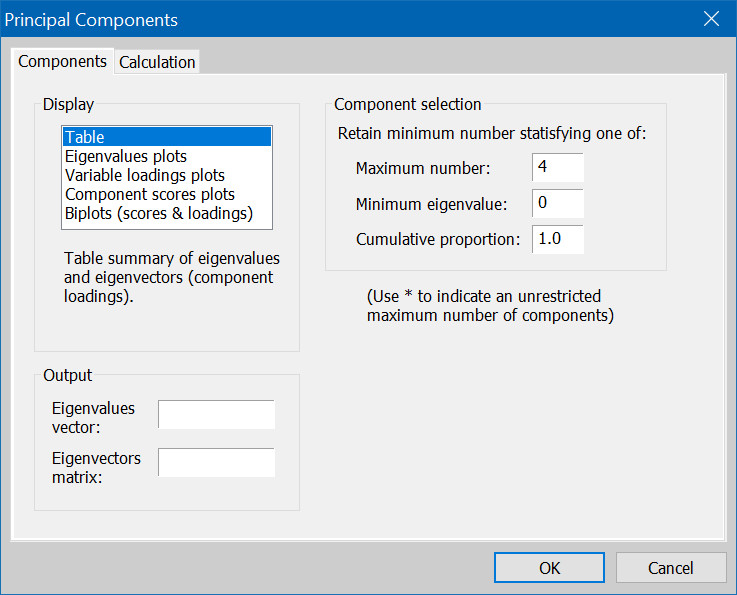Variable loading plots produce $XY$ ''-pair plots of loading vectors. See section Loading Plots in Part I of this series for further details. The user specifies which loading vectors to compare and selects one among the following loading (scaling) protocols:
• Normalize Scores: Here, the scaling factor is the square root of the eigenvalue vector. In other words, the $k^{\text{th}}$ element of the $i^{\text{th}}$ loading vector is the $k^{\text{th}}$ element of the $i^{\text{th}}$ eigenvector, multiplied by the square root of the $k^{\text{th}}$ eigenvalue.
• Symmetric Weights: In this scenario, the scaling factor is the quartic (fourth) root of the eigenvalue vector. Namely, the $k^{\text{th}}$ element of the $i^{\text{th}}$ loading vector is the $k^{\text{th}}$ element of the $i^{\text{th}}$ eigenvector, multiplied by the fourth root of the $k^{\text{th}}$ eigenvalue.
• User Loading Weight: If $0 \leq \omega \leq 1$ denotes the user defined scaling factor, then the loading vectors are formed by scaling the $k^{\text{th}}$ element of the corresponding eigenvector by the $k^{\text{th}}$ eigenvalue raised to the power $\omega/2$ .
For the time being, stick with all default values. That is, we will look at the loading plots across the first two principal directions, and we will use the Normalize Loadings scaling protocol. In other words, we will plot the true eigenvectors since scaling is unity. Note that the choice of looking at only the first two principal directions is, among other things, motivated by our previous analysis on dimension reduction where we decided to retain only the first two principal eigenvalues and discard the rest. Go ahead and click on OK. Figure 10 summarizes the output.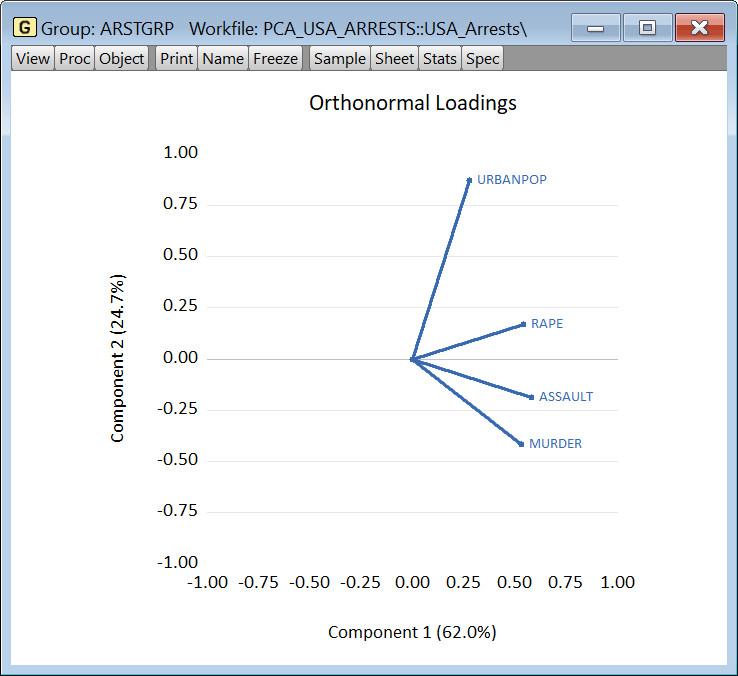As discussed in section Loading Plots in Part I of this series, the angle between the vectors in a loading plot is related to the correlation between the original variables to which the loading vectors are associated. Accordingly, we see that MURDER and ASSAULT are moderately positively correlated, as are ASSAULT and RAPE, although the latter two less so than the former two. Moreover, it is clear that RAPE and URBANPOP are positively correlated, whereas MURDER and URBANPOP are nearly uncorrelated since they form a near 90 degree angle. In other words, we have a two-dimensional graphical representation of the four-dimensional correlation matrix in Figure 4b. This ability to represent higher dimensional information in a lower dimensional space is arguably the most useful feature of PCA.

Furthermore, all three variables, MURDER, ASSAULT, and RAPE, are strongly correlated with the first principal direction, whereas URBANPOP is strongly correlated with the second principal direction. In fact, looking at vector lengths, we can also see that MURDER, ASSAULT, and RAPE are roughly equally dominant in the first direction, whereas URBANPOP is significantly more dominant than either of the former three, albeit in the second direction. Of course, this simply confirms our preliminary analysis of the middle table in Figure 6.

Above, we started with the basic loading vector with scale unity. We could have, of course, resorted to other scaling options such as normalizing to the score vectors, using symmetric weights, or using some other custom weighting. Since each of these would yield a different but similar perspective, we won't delve further into details. Nevertheless, as an exercise in exhibiting the steps involved, we provide below small snippets of code to manually generate loading vectors using only the eigenvalues and eigenvectors associated with the underlying correlation matrix. This is done for each of the four scaling protocols. These manually generated vectors are then compared to the loading vectors generated by EViews' internal code and shown to be identical.

 ' --- Verify Loading Plot Vectors ---
group crime murder assault rape urbanpop ' create group with all 4 variables

' make eigenvalues and eigenvectors based on the corr. matrix
crime.pcomp(eigval=eval, eigvec=evec, cov=corr)

matrix evaldiag = @makediagonal(eval) ' create diagonal matrix of eigenvalues

'normalize scores

'symmetric weights

'user weights


#### Score Analysis

Whereas loading vectors reveal information on which variables dominate (and by how much) each principal direction, it is only when they are used to create the principal component vectors (score vectors) that they are truly useful in a data exploratory sense. In this regard, we again open the main principal component dialog and select Component scores plots in the Display group of options. We capture this in Figure 11 below.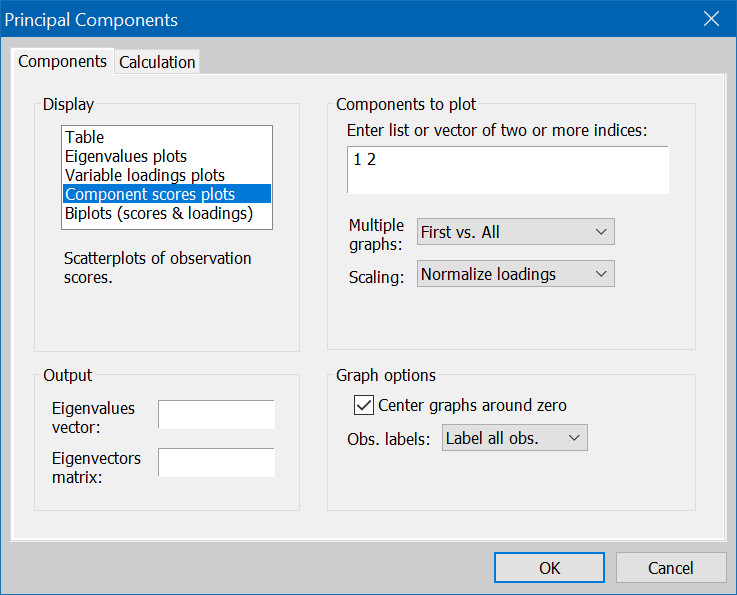Figure 11: PCA Dialog: Component Scores Plots

Analogous to the loading vector plots, here, EViews produces $XY$ ''-pair plots of score vectors. As in the case of loading plots, the user specifies which score vectors to compare, and selects one among the following loading (scaling) protocols:
• Normalize Loadings: Score vectors are scaled by unity. In other words, no scaling occurs.
• Normalize Scores: The $k^{\text{th}}$ score vector is scaled by the inverse of the square root of the $k^{\text{th}}$ eigenvalue.
• Symmetric Weights: The $k^{\text{th}}$ score vector is scaled by the inverse of the quartic root of the $k^{\text{th}}$ eigenvalue.
• User Loading Weight: If $0 \leq \omega \leq 1$ denotes the user defined scaling factor, the $k^{\text{th}}$ score vector is scaled by the $k^{\text{th}}$ eigenvalue raised to the power $-\omega/2$.
Furthermore, if outlier detection is desired, EViews allows users to specify a p-value as a detection threshold. See sections Score Plots and Outlier Detection in Part I of this series for further details. Since we are currently interested in interpretive exercises, we will forgo outlier detection and choose to display all observations. To do so, under the Graph options group of options, change the Obs. Labels to Label all obs. and hit OK. We replicate the output in Figure 12.Figure 12: Component Scores Plots Output

The output produced is a scatter plot of principal component 1 (score vector 1) vs. principal component 2 (score vector 2). There are several important observations to be made here.

First, the further east of the zero vertical axis a state is located, the more positively correlated it is with the first principal direction. Since the latter is dominated positively (east of the zero vertical axis) by the three crime categories MURDER, ASSAULT, and RAPE (see Figure 11), we conclude that such states are positively correlated with said crimes. Naturally, converse conclusions hold as well. In particular, we see that CALIFORNIA, NEVADA, and FLORIDA are most positively correlated with the three crimes under consideration. If this is indeed the case, then it is little surprise that most Hollywood productions typically involve crime thrillers set in these three states. Conversely, NORTH DAKOTA and VERMONT are typically least associated with the crimes under consideration.

Second, the further north of the zero horizontal axis a state is located, the more positively correlated it is with the second principal direction. Since the latter is dominated positively (north of the zero horizontal axis) by the variable URBNAPOP (see Figure 11), we conclude that such states are positively correlated with urbanization. Again, the converse conclusions hold as well. In particular, HAWAII, CALIFORNIA, RHODE ISLAND, MASSACHUSETTS, UTAH, NEW JERSEY are states most positively associated with urbanization, whereas those least so are SOUTH CAROLINA, NORTH CAROLINA, and MISSISSIPPI.

Lastly, it is worth recalling that like loading vectors, score vectors can also be scaled. In this regard, we provide code snippets below to show how to manually compute scaled score vectors, exposing the algorithm that EViews uses to do same in its internal computations.

 ' --- Verify Score Vectors ---
' make eigenvalues and eigenvectors based on the corr. matrix
crime.pcomp(eigval=eval, eigvec=evec, cov=corr)

matrix evaldiag = @makediagonal(eval) ' create diagonal matrix of eigenvalues

stom(crime, crimemat) ' create matrix from crime group
vector means = @cmean(crimemat) ' get column means
vector popsds = @cstdevp(crimemat) ' get population standard deviations

' initialize matrix for normalized crimemat
matrix(@rows(crimemat), @columns(crimemat)) crimematnorm

' normalize (remove mean and divide by pop. s.d.) every column of crimemat
for !k = 1 to @columns(crimemat)
colplace(crimematnorm,(@columnextract(crimemat,!k) - means(!k))/popsds(!k),!k)
next

crime.makepcomp(cov=corr) s1 s2 s3 s4 ' get score series
group scores s1 s2 s3 s4 ' put scores into group
stom(scores, scoremat) ' put scores group into matrix
matrix scoreverify = crimematnorm*evec ' create custom score matrix
matrix scorediff = scoreverify - scoremat ' get difference between custom and eviews output
show scorediff

'normalize scores
crime.makepcomp(scale=normscores, cov=corr) s1 s2 s3 s4
group scores s1 s2 s3 s4
stom(scores, scoremat)
scoreverify = crimematnorm*evec*@inverse(@epow(evaldiag, 0.5))
scorediff = scoreverify - scoremat
show scorediff

'symmetric weights
crime.makepcomp(scale=symmetrics, cov=corr) s1 s2 s3 s4
group scores s1 s2 s3 s4
stom(scores, scoremat)
scoreverify = crimematnorm*evec*@inverse(@epow(evaldiag, 0.25))
scorediff = scoreverify - scoremat
show scorediff

'user weights
crime.makepcomp(scale=0.36, cov=corr) s1 s2 s3 s4
group scores s1 s2 s3 s4
stom(scores, scoremat)
scoreverify = crimematnorm*evec*@inverse(@epow(evaldiag, 0.18))
scorediff = scoreverify - scoremat
show scorediff

Above, observe that we derived eigenvalues and eigenvectors of the correlation matrix. Accordingly, to derive the score vectors manually, we needed to standardize the original variables first. In this regard, when using the covariance matrix instead, one need only to demean the original variables and disregard scaling information. We leave this as an exercise to interested readers.

#### Biplot Analysis

As a last exercise, we superimpose the loading vectors and score vectors onto a single graph called the biplot. To do this, again, bring up the main principal component dialog and under the Display group select Biplot (scores & loadings). As in the previous exercise, under the Graph options group, select Label all obs. from the Obs. labels dropdwon, and hit OK. We summarize these steps in Figure 13.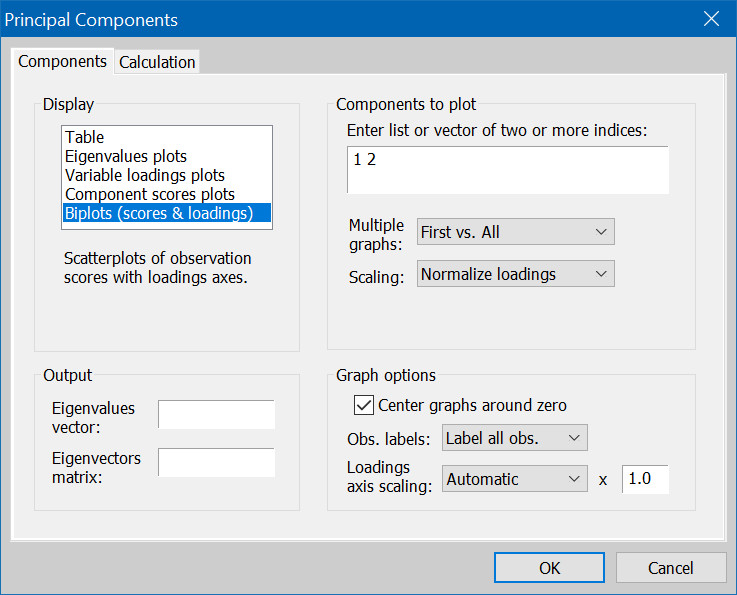From an inferential standpoint, there's little to contribute beyond what we laid out in each of the previous two sections. Nevertheless, having both the loading and score vectors appear on the same graph visually reinforces our previous analysis. Accordingly, we close this section with just the graphical output.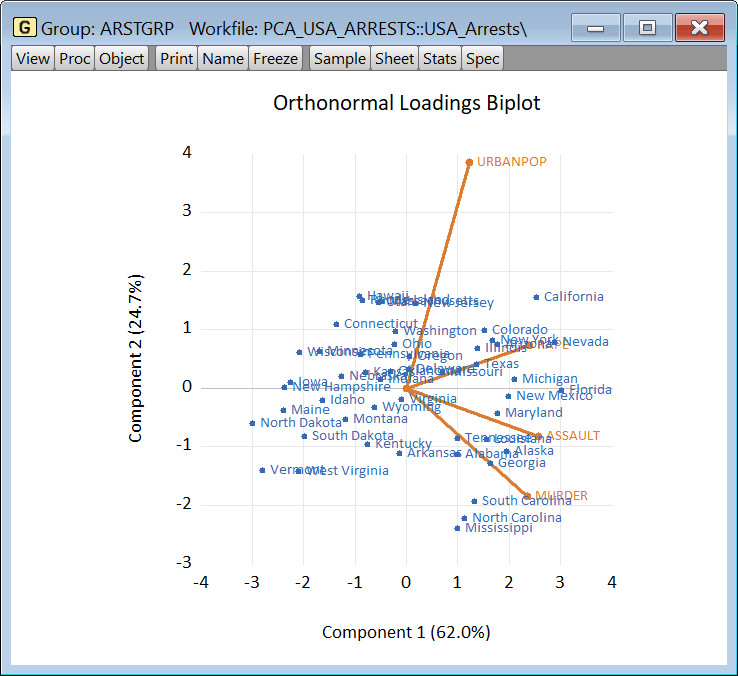### Concluding Remarks

In Part I of this series we laid out the theoretical foundations underlying PCA. Here, we used EViews to conduct a brief data exploratory implementation of PCA on serious crimes across 50 US states. Our aim was to illustrate the use of numerous PCA tools available in EViews with brief interpretations associated with each.

In closing, we would like to point out that apart from the main principal component dialog we used above, EViews also offers a Make Principal Components... proc function which provides a unified framework for producing vectors and matrices of the most important objects related to PCA. These include the vector of eigenvalues, the matrix of eigenvectors, the matrix of loading vectors, as well as the matrix of scores. To access this function, open the crime group from the workfile, click on Proc and click on Make Principal Components.... We summarize this in Figures 15a and 15b below.Figure 15a: Group Proc: Make Principal Components... Figure 15b: Make Principal Components Dialog
From here, one can insert names for all objects one wishes to place in the workfile, select the scaling one wishes to use in the creation of the loading and score vectors, and hit OK.

### Files

1.